Ex 9.2

Chapter 9 Class 11 Sequences and Series
Serial order wise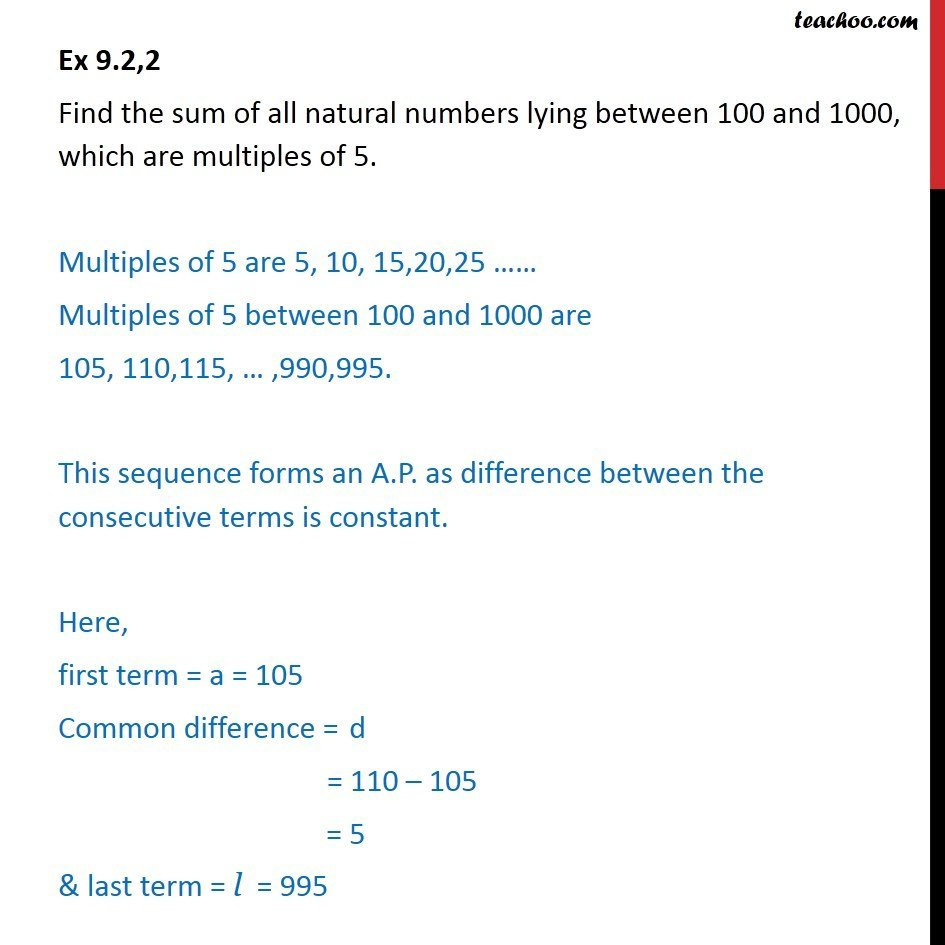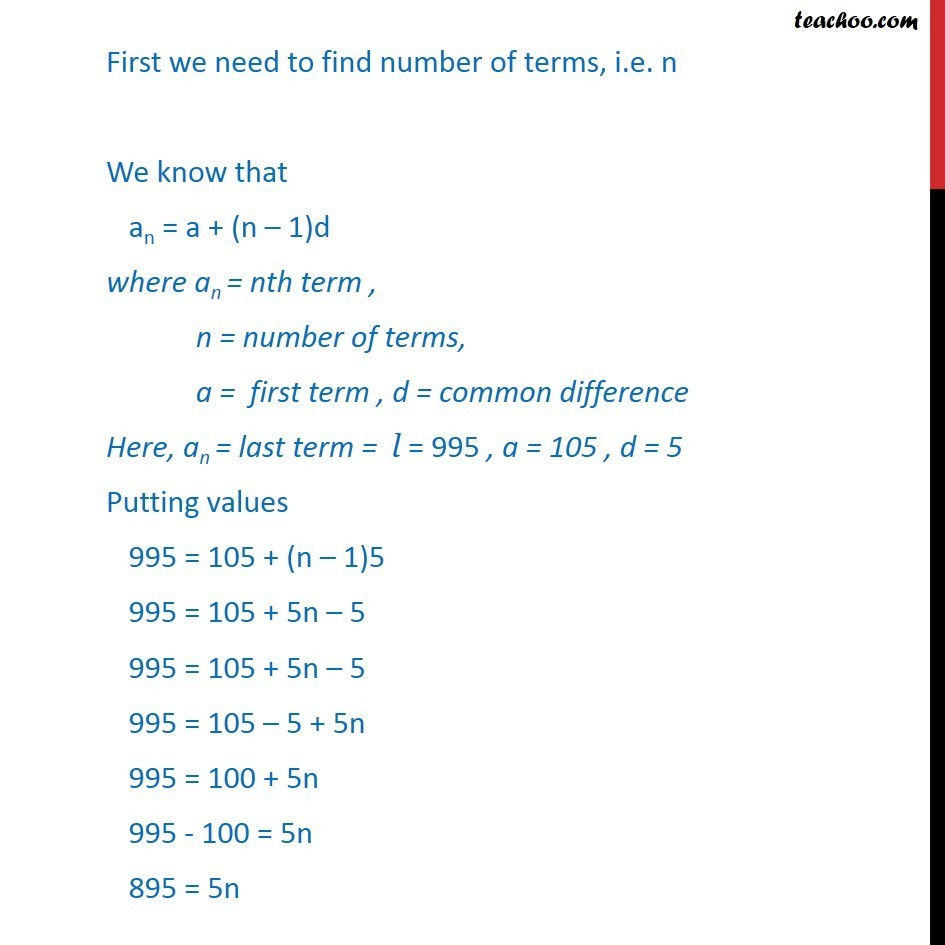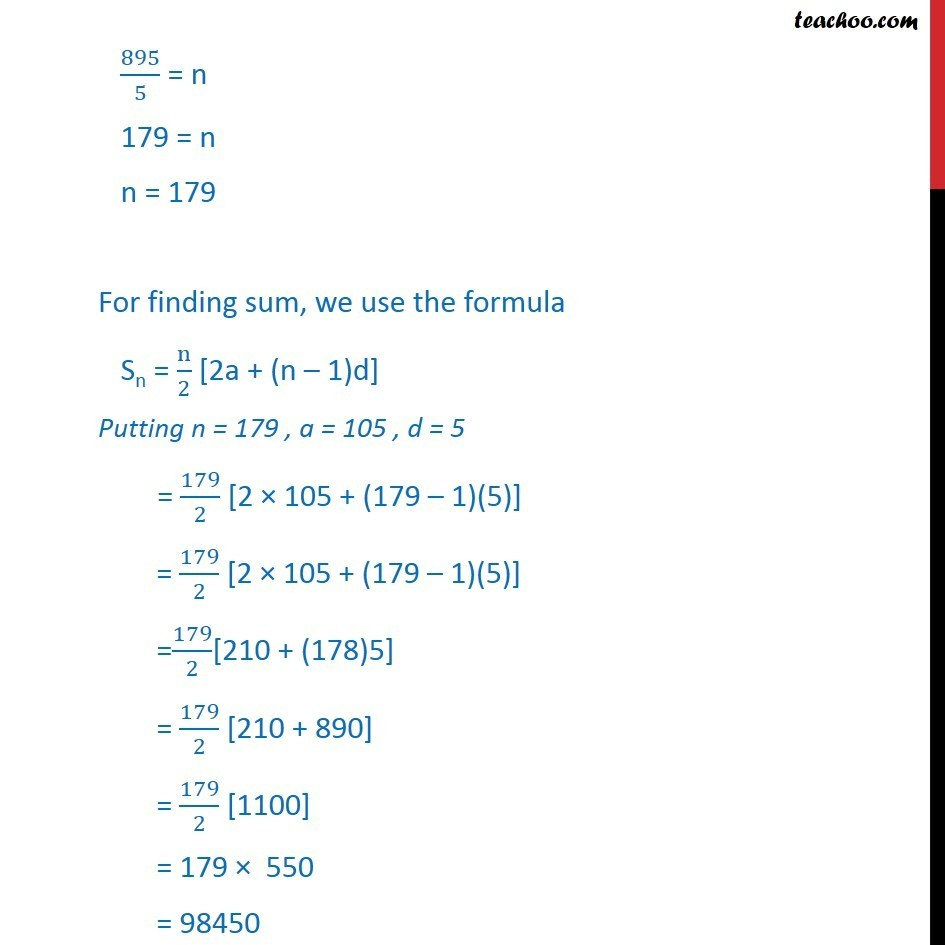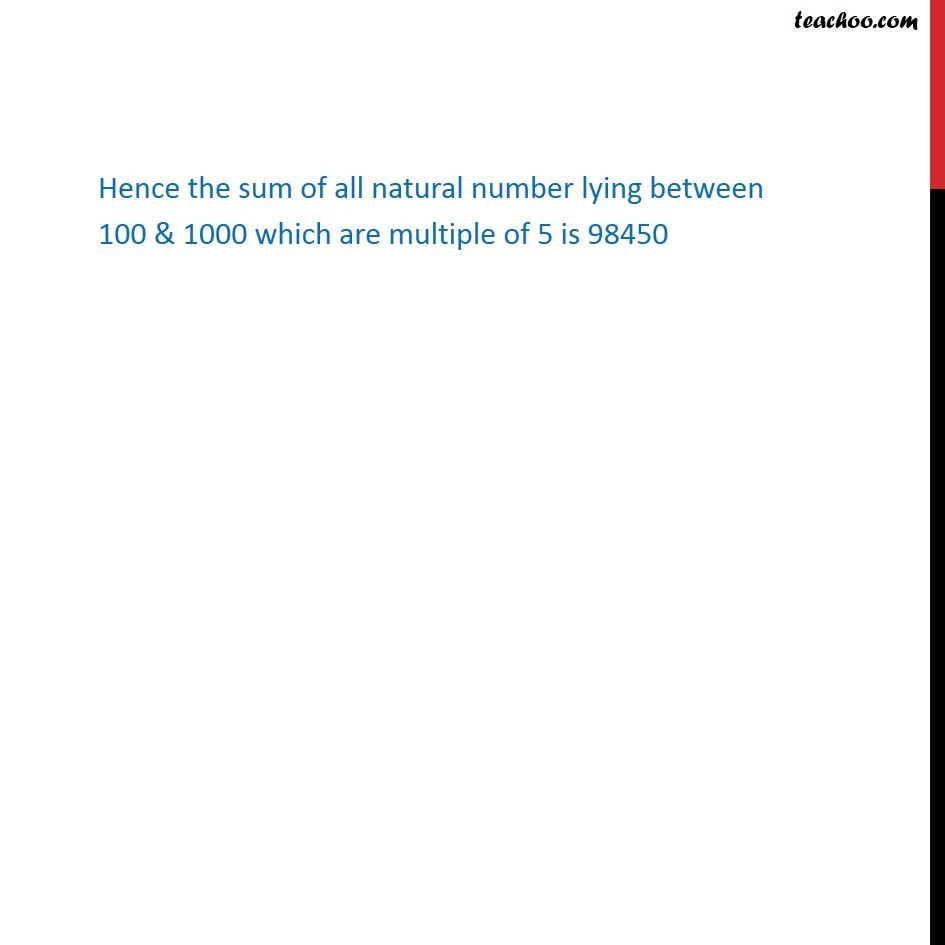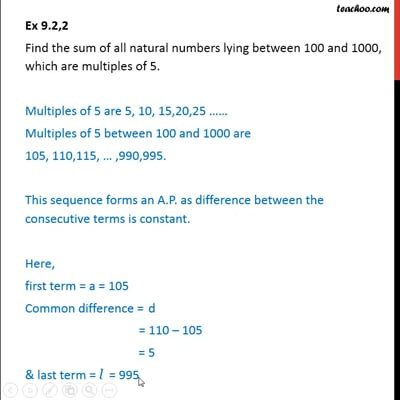This video is only available for Teachoo black users

Solve all your doubts with Teachoo Black (new monthly pack available now!)

### Transcript

Ex 9.2,2 Find the sum of all natural numbers lying between 100 and 1000, which are multiples of 5. Multiples of 5 are 5, 10, 15,20,25 …… Multiples of 5 between 100 and 1000 are 105, 110,115, … ,990,995. This sequence forms an A.P. as difference between the consecutive terms is constant. Here, first term = a = 105 Common difference = d = 110 – 105 = 5 & last term = l = 995 First we need to find number of terms, i.e. n We know that an = a + (n – 1)d where an = nth term , n = number of terms, a = first term , d = common difference Here, an = last term = l = 995 , a = 105 , d = 5 Putting values 995 = 105 + (n – 1)5 995 = 105 + 5n – 5 995 = 105 + 5n – 5 995 = 105 – 5 + 5n 995 = 100 + 5n 995 - 100 = 5n 895 = 5n 895/5 = n 179 = n n = 179 For finding sum, we use the formula Sn = n/2 [2a + (n – 1)d] Putting n = 179 , a = 105 , d = 5 = 179/2 [2 × 105 + (179 – 1)(5)] = 179/2 [2 × 105 + (179 – 1)(5)] =179/2[210 + (178)5] = 179/2 [210 + 890] = 179/2  = 179 × 550 = 98450 Hence the sum of all natural number lying between 100 & 1000 which are multiple of 5 is 98450